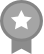```Hello! I'm Grim! I write whenever I want, and I draw most of my covers for my story's. Hood y'all enjoy it here!

Edit: I've been on this app for 4 years, it's been great, but lately I've started to notice that wattpad has become less of a website or and app that u can enjoy now, cause it no longer values it readers and writers. All it seems to care about is the money now.

∧_∧
( ･∀･)
⊂ ⊂　)
( (  (
(_(＿)

Good
∧_∧
( ･∀･)
( Ｕ   つ
)  )  )
(＿)_)
So
∧_∧
（ ･∀･)
⊂_へ　つ
(＿)｜

I'm Ganna
∧_∧
(･_･)っ
(っ /
Lﾉ┘
Follow
∧___∧
⊂(・＿・ )
ヽ ⊂二/
(⌒)  /

You!
／　　　　　　　 ＼
|　　●　　　　●      |
＼　       _

(•_•)
/    \

( •_•)
<(   (>  Come and get it
/    \

(•_•)
~(   )~  NA NA NA NA
/    \"

(•_•)
<)   )- if yo age is 1+
/    \

(•_•)
/(   (> on the this day , get
/    \

(•_•)/
<)   ) ready for this book
/    \

\(•_•)
/ \

(•_•)
<) . )/  well
/  \

\(•_•)
( . (> then
/ \

(•_•)
<) . )>  bye
/  \

\(•_•)
( . (> so I'm like
/ \

(╯°□°)╯︵ ┻━┻ FLIP THAT TABLE.

┻━┻ ︵ ヽ(°□°ヽ) FLIP THIS TABLE.

┻━┻ ︵ ＼('0')/／ ︵ ┻━┻ FLIP ALL THE TABLES

ಠ_ಠ Child. . .

ಠ_ಠ Put.

ಠ__ಠ The tables.

ಠ___ಠ Back.

(╮°-°)╮┳━┳

(╯°□°)╯︵ ┻━┻ NEVER

(•_•)
<)   )╯because I'm
/    \

⊂_ヽ
＼＼ ＿
＼(　•_•) F
<　⌒ヽ A
/ 　 へ＼ B
/　　/　＼＼ U
ﾚ　ノ　　 ヽ_つ L
/　/                   O
/　/|                   U
(　(ヽ               S
|　|    \__
| 丿 ＼ ⌒)
| |　　) /
'ノ )　   Lﾉ
(_／(totally didn't copy this).

• In Another Dimension
• JoinedMarch 30, 2017

Following#452 in skeleton See all rankings